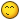# Weird function

## Recommended Posts

I found this function in the code and one of the following things is true:
* this function is wrong

* I'm being thick

Phaser.Point.rotate = function (a, x, y, angle, asDegrees, distance) {

asDegrees = asDegrees || false;
distance = distance || null;

if (asDegrees)
{
}

//  Get distance from origin (cx/cy) to this point
if (distance === null)
{
distance = Math.sqrt(((x - a.x) * (x - a.x)) + ((y - a.y) * (y - a.y)));
}

return a.setTo(x + distance * Math.cos(angle), y + distance * Math.sin(angle));

};

From the description in the docs, I expected the point (a.x, a.y) to be rotated around (x, y) by angle.
More explicitly, something like:

// Cast

// Translate so the rotation is about the origin
var tx = a.x - x;
var ty = a.y - y;

// Rotate
return a.setTo(Math.cos(angle) * tx - Math.sin(angle) * ty + x, Math.sin(angle) * tx + Math.cos(angle) * ty + y);

That said, I don't know what the "distance" parameter is supposed to be doing, so I've probably just misunderstood this function :-/.

Edit: and to all of those doing the Ludum Dare (like I am), I hope you're having as much fun as me :-D! Phaser is really making this a smooth experience - loving it so far.